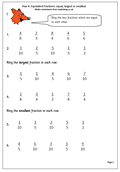# Resource of the week: Equivalent fractionsOnce the idea of equivalent fractions has been understood it becomes possible to compare two fractions to see which is the larger. The first thing to remember is that the larger the number on the bottom of the fraction, the smaller each part of the fraction is. So 4/100 is much smaller than 4/25.

Now this is also easy if the bottom number (denominator) is the same in each fraction eg 1/5 is smaller than 3/5.

The difficulty comes when the numbers are not the same. How do you compare 3/5 with 7/10?

The easiest way is to make the bottom number of each fraction the same, and in the case above this will mean converting the 3/5 into tenths. We can do this by multiplying the 5 by 2 to make 10 and we have to do the same with the top number, multiplying the 3 by 2. In this way 3/5 can be converted to 6/10.

3/5 and 6/10 are equal, or equivalent.

It is now easy to see that 6/10 is smaller than 7/10, so 3/5 must also be smaller than 7/10. Job done!

Later it will become harder to convert so that the denominators are the same; sometimes you have to multiply both fractions by different numbers, but this comes later! At the moment it is important to get the basics correct.

Equivalent fractions: comparing